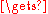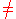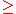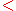xBrute-force searchEncyclopedia
In computer science
Computer science
Computer science or computing science is the study of the theoretical foundations of information and computation and of practical techniques for their implementation and application in computer systems...

, brute-force search or exhaustive search, also known as generate and test, is a trivial but very general problem-solving technique that consists of systematically enumerating all possible candidates for the solution and checking whether each candidate satisfies the problem's statement. For example, a brute-force algorithm to find the divisor
Divisor
In mathematics, a divisor of an integer n, also called a factor of n, is an integer which divides n without leaving a remainder.-Explanation:...

s of a natural number
Natural number
In mathematics, the natural numbers are the ordinary whole numbers used for counting and ordering . These purposes are related to the linguistic notions of cardinal and ordinal numbers, respectively...

n is to enumerate all integers from 1 to the square-root of n, and check whether each of them divides n without remainder. For another example, consider the popular eight queens puzzle
Eight queens puzzle
The eight queens puzzle is the problem of placing eight chess queens on an 8×8 chessboard so that no two queens attack each other. Thus, a solution requires that no two queens share the same row, column, or diagonal...

, which asks to place eight queen
Queen (chess)
The queen is the most powerful piece in the game of chess, able to move any number of squares vertically, horizontally, or diagonally. Each player starts the game with one queen, placed in the middle of the first rank next to the king. With the chessboard oriented correctly, the white queen starts...

s on a standard chessboard
Chessboard
A chessboard is the type of checkerboard used in the board game chess, and consists of 64 squares arranged in two alternating colors...

so that no queen attacks any other. A brute-force approach would examine all possible arrangements of 8 pieces in the 64 squares, and, for each arrangement, check whether any queen attacks any other. Brute-force search is simple to implement, and will always find a solution if it exists. However, its cost is proportional to the number of candidate solutions, which, in many practical problems, tends to grow very quickly as the size of the problem increases. Therefore, brute-force search is typically used when the problem size is limited, or when there are problem-specific heuristics that can be used to reduce the set of candidate solutions to a manageable size. The method is also used when the simplicity of implementation is more important than speed. This is the case, for example, in critical applications where any errors in the algorithm would have very serious consequences; or when using a computer to prove a mathematical theorem
Automated theorem proving
Automated theorem proving or automated deduction, currently the most well-developed subfield of automated reasoning , is the proving of mathematical theorems by a computer program.- Decidability of the problem :...

. Brute-force search is also useful as "baseline" method when benchmarking
Benchmarking
Benchmarking is the process of comparing one's business processes and performance metrics to industry bests and/or best practices from other industries. Dimensions typically measured are quality, time and cost...

other algorithms or metaheuristic
Metaheuristic
In computer science, metaheuristic designates a computational method that optimizes a problem by iteratively trying to improve a candidate solution with regard to a given measure of quality. Metaheuristics make few or no assumptions about the problem being optimized and can search very large spaces...

s. Indeed, brute-force search can be viewed as the simplest metaheuristic. Brute force search should not be confused with backtracking
Backtracking
Backtracking is a general algorithm for finding all solutions to some computational problem, that incrementally builds candidates to the solutions, and abandons each partial candidate c as soon as it determines that c cannot possibly be completed to a valid solution.The classic textbook example...

, where large sets of solutions can be discarded without being explicitly enumerated (as in the textbook computer solution to the eight queens problem above). The brute-force method for finding an item in a table — namely, check all entries of the latter, sequentially — is called linear search
Linear search
In computer science, linear search or sequential search is a method for finding a particular value in a list, that consists of checking every one of its elements, one at a time and in sequence, until the desired one is found....

.

### Basic algorithm

In order to apply brute-force search to a specific class of problems, one must implement four procedures
Subroutine
In computer science, a subroutine is a portion of code within a larger program that performs a specific task and is relatively independent of the remaining code....

, first,next, valid, and output. These procedures should take as a parameter the data P for the particular instance of the problem that is to be solved, and should do the following:
1. first (P): generate a first candidate solution for P.
2. next (P, c): generate the next candidate for P after the current one c.
3. valid (P, c): check whether candidate c is a solution for P.
4. output (P, c): use the solution c of P as appropriate to the application.

The next procedure must also tell when there are no more candidates for the instance P, after the current one c. A convenient way to do that is to return a "null candidate", some conventional data value Λ that is distinct from any real candidate. Likewise the first procedure should return Λ if there are no candidates at all for the instance P. The brute-force method is then expressed by the algorithm
cfirst(P)
while cΛ do if valid(P,c) then output(P, c) cnext(P,c)
For example, when looking for the divisors of an integer n, the instance data P is the number n. The call first(n) should return the integer 1 if n1, or Λ otherwise; the call next(n,c) should return c + 1 if cn, and Λ otherwise; and valid(n,c) should return true if and only if c is a divisor of n. (In fact, if we choose Λ to be n + 1, the tests n1 and cn are unnecessary.)The brute-force search algorithm above will call output for every candidate that is a solution to the given instance P. The algorithm is easily modified to stop after finding the first solution, or a specified number of solutions; or after testing a specified number of candidates, or after spending a given amount of CPU
Central processing unit
The central processing unit is the portion of a computer system that carries out the instructions of a computer program, to perform the basic arithmetical, logical, and input/output operations of the system. The CPU plays a role somewhat analogous to the brain in the computer. The term has been in...

time.

## Combinatorial explosion

The main disadvantage of the brute-force method is that, for many real-world problems, the number of natural candidates is prohibitively large. For instance, if we look for the divisors of a number as described above, the number of candidates tested will be the given number n. So if n has sixteen decimal digits, say, the search will require executing at least 1015 computer instructions, which will take several days on a typical PC
Personal computer
A personal computer is any general-purpose computer whose size, capabilities, and original sales price make it useful for individuals, and which is intended to be operated directly by an end-user with no intervening computer operator...

. If n is a random 64-bit natural number, which has about 19 decimal digits on the average, the search will take about 10 years. This steep growth in the number of candidates, as the size of the data increases, occurs in all sorts of problems. For instance, if we are seeking a particular rearrangement of 10 letters, then we have 10! = 3,628,800 candidates to consider, which a typical PC can generate and test in less than one second. However, adding one more letter — which is only a 10% increase in the data size — will multiply the number of candidates by 11 — a 1000% increase. For 20 letters, the number of candidates is 20!, which is about 2.4×1018 or 2.4 quintillion; and the search will take about 10,000 years. This unwelcome phenomenon is commonly called the combinatorial explosion
Combinatorial explosion
In administration and computing, a combinatorial explosion is the rapidly accelerating increase in lines of communication as organizations are added in a process...

.

## Speeding up brute-force searches

One way to speed up a brute-force algorithm is to reduce the search space, that is, the set of candidate solutions, by using heuristic
Heuristic
Heuristic refers to experience-based techniques for problem solving, learning, and discovery. Heuristic methods are used to speed up the process of finding a satisfactory solution, where an exhaustive search is impractical...

s specific to the problem class.
For example, consider the popular eight queens problem, which asks to place eight queen
Queen (chess)
The queen is the most powerful piece in the game of chess, able to move any number of squares vertically, horizontally, or diagonally. Each player starts the game with one queen, placed in the middle of the first rank next to the king. With the chessboard oriented correctly, the white queen starts...

s on a standard chessboard
Chessboard
A chessboard is the type of checkerboard used in the board game chess, and consists of 64 squares arranged in two alternating colors...

so that no queen attacks any other. Since each queen can be placed in any of the 64 squares, in principle there are 648 = 281,474,976,710,656 (over 281 trillion) possibilities to consider. However, if we observe that the queens are all alike, and that no two queens can be placed on the same square, we conclude that the candidates are all possible ways of choosing of a set of 8 squares from the set all 64 squares; which means 64 choose 8 = 64!/56!8! = 4,426,165,368 candidate solutions — about 1/60,000 of the previous estimate. Actually, it is easy to see that no arrangement with two queens on the same row or the same column can be a solution. Therefore, we can further restrict the set of candidates to those arrangements where queen 1 is in row 1, queen 2 is in row 2, and so on; all in different columns. We can describe such an arrangement by an array of eight numbers c through c, each of them between 1 and 8, where c is the column of queen 1, c is the column of queen 2, and so on. Since these numbers must be all different, the number of candidates to search is the number of permutation
Permutation
In mathematics, the notion of permutation is used with several slightly different meanings, all related to the act of permuting objects or values. Informally, a permutation of a set of objects is an arrangement of those objects into a particular order...

s of the integers 1 through 8, namely 8! = 40,320 — about 1/100,000 of the previous estimate, and 1/7,000,000,000 of the first one. As this example shows, a little bit of analysis will often lead to dramatic reductions in the number of candidate solutions, and may turn an intractable problem into a trivial one. This example also shows that the candidate enumeration procedures (first and next) for the restricted set of candidates may be just as simple as those of the original set, or even simpler.In some cases, the analysis may reduce the candidates to the set of all valid solutions; that is, it may yield an algorithm that directly enumerates all the desired solutions (or finds one solution, as appropriate), without wasting time with tests and the generation of invalid candidates. For example, consider the problem of finding all integers between 1 and 1,000,000 that are evenly divisible by 417. A naive brute-force solution would generate all integers in the range, testing each of them for divisibility. However, that problem can be solved much more efficiently by starting with 417 and repeatedly adding 417 until the number exceeds 1,000,000 — which takes only 2398 (= 1,000,000 ÷ 417) steps, and no tests.

## Reordering the search space

In applications that require only one solution, rather than all solutions, the expected
Expected value
In probability theory, the expected value of a random variable is the weighted average of all possible values that this random variable can take on...

running time of a brute force search will often depend on the order in which the candidates are tested. As a general rule, one should test the most promising candidates first. For example, when searching for a proper divisor of a random number n, it is better to enumerate the candidate divisors in increasing order, from 2 to n - 1, than the other way around — because the probability that n is divisible by c is 1/c.Moreover, the probability of a candidate being valid is often affected by the previous failed trials. For example, consider the problem of finding a 1 bit in a given 1000-bit string P. In this case, the candidate solutions are the indices 1 to 1000, and a candidate c is valid if P[c] = 1. Now, suppose that the first bit of P is equally likely to be 0 or 1, but each bit thereafter is equal to the previous one with 90% probability. If the candidates are enumerated in increasing order, 1 to 1000, the number t of candidates examined before success will be about 6, on the average. On the other hand, if the candidates are enumerated in the order 1,11,21,31...991,2,12,22,32 etc., the expected value of t will be only a little more than 2.More generally, the search space should be enumerated in such a way that the next candidate is most likely to be valid, given that the previous trials were not. So if the valid solutions are likely to be "clustered" in some sense, then each new candidate should be as far as possible from the previous ones, in that same sense. The converse holds, of course, if the solutions are likely to be spread out more uniformly than expected by chance.

## Alternatives to brute force search

There are many other search methods, or metaheuristics, which are designed to take advantage of various kinds of partial knowledge one may have about the solution. Heuristic
Heuristic
Heuristic refers to experience-based techniques for problem solving, learning, and discovery. Heuristic methods are used to speed up the process of finding a satisfactory solution, where an exhaustive search is impractical...

s can also be used to make an early cutoff of parts of the search. One example of this is the minimax
Minimax
Minimax is a decision rule used in decision theory, game theory, statistics and philosophy for minimizing the possible loss for a worst case scenario. Alternatively, it can be thought of as maximizing the minimum gain...

principle for searching game trees, that eliminates many subtrees at an early stage in the search. In certain fields, such as language parsing, techniques such as chart parsing can exploit constraints in the problem to reduce an exponential complexity problem into a polynomial complexity problem. In many cases, such as in Constraint Satisfaction Problem
Constraint satisfaction problem
Constraint satisfaction problems s are mathematical problems defined as a set of objects whose state must satisfy a number of constraints or limitations. CSPs represent the entities in a problem as a homogeneous collection of finite constraints over variables, which is solved by constraint...

s, one can dramatically reduce the search space by means of Constraint propagation, that is efficiently implemented in Constraint programming
Constraint programming
Constraint programming is a programming paradigm wherein relations between variables are stated in the form of constraints. Constraints differ from the common primitives of imperative programming languages in that they do not specify a step or sequence of steps to execute, but rather the properties...

languages.The search space for problems can also be reduced by replacing the full problem with a simplified version. For example, in computer chess
Computer chess
Computer chess is computer architecture encompassing hardware and software capable of playing chess autonomously without human guidance. Computer chess acts as solo entertainment , as aids to chess analysis, for computer chess competitions, and as research to provide insights into human...

, rather than computing the full minimax
Minimax
Minimax is a decision rule used in decision theory, game theory, statistics and philosophy for minimizing the possible loss for a worst case scenario. Alternatively, it can be thought of as maximizing the minimum gain...

tree of all possible moves for the remainder of the game, a more limited tree of minimax possibilities is computed, with the tree being pruned at a certain number of moves, and the remainder of the tree being approximated by a static evaluation function
Evaluation function
An evaluation function, also known as a heuristic evaluation function or static evaluation function, is a function used by game-playing programs to estimate the value or goodness of a position in the minimax and related algorithms...

.

## In cryptography

In cryptography
Cryptography
Cryptography is the practice and study of techniques for secure communication in the presence of third parties...

, a brute-force attack involves systematically checking all possible keys
Key (cryptography)
In cryptography, a key is a piece of information that determines the functional output of a cryptographic algorithm or cipher. Without a key, the algorithm would produce no useful result. In encryption, a key specifies the particular transformation of plaintext into ciphertext, or vice versa...

until the correct key is found. This strategy
Strategy
Strategy, a word of military origin, refers to a plan of action designed to achieve a particular goal. In military usage strategy is distinct from tactics, which are concerned with the conduct of an engagement, while strategy is concerned with how different engagements are linked...

can in theory be used against any encrypted data by an attacker who is unable to take advantage of any weakness in an encryption system that would otherwise make his task easier.

The key length used in the encryption determines the practical feasibility of performing a brute force attack, with longer keys exponentially more difficult to crack than shorter ones. Brute force attacks can be made less effective by obfuscating
Obfuscation
Obfuscation is the hiding of intended meaning in communication, making communication confusing, wilfully ambiguous, and harder to interpret.- Background :Obfuscation may be used for many purposes...

the data to be encoded, something that makes it more difficult for an attacker to recognise when he has cracked the code. One of the measures of the strength of an encryption system is how long it would theoretically take an attacker to mount a successful brute force attack against it.